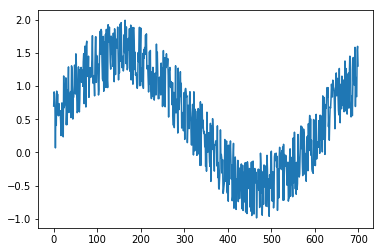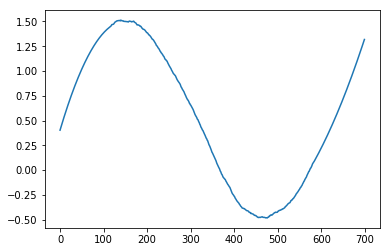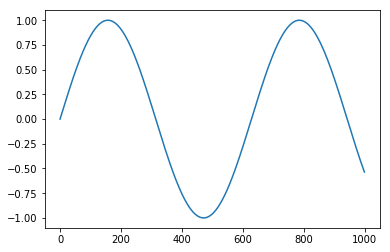# Applying XND on your data analysis workflow¶

This document show some ways to apply XND on a data analysis workflow.

Basically, a data analysis workflow has 5 main task groups:

• domain acknolodge
• data storage
• data cleaning
• data processing
• data visualization

This document focus on the last 3 task groups: data cleaning, processing and visualization.

Some examples will be discussed using xnd with gumath integrated with other libraries

## Setup¶

First we need to import some libraries

In :
import sys

In :
from importnb import Notebook
from IPython.display import display
import random
from xnd import xnd

In :
xnd_gumath = Notebook.load('utils/math_utils.ipynb', main=True).xnd_gumath


## Data Process¶

The data processing tasks aim to work on raw/cleaning data and get information from that. Digital Signal Processing, Machine Learning, Math, Statistics, Scientific Computing, etc are some possible approaches that someone want to work with data. This document shows some operations with: Math

### Math operations¶

XND math operations could be done using gumath. The follow examples use a class that combine xnd and gumath libraries (@ xnd_gumath). libgumath is an C library that supports a general dispatch mechanism for xnd containers as well as a composable, generalized function concept.

Binary operations:

In :
# using arrays from a dictionary
v = xnd_gumath({'x': [1, 2, 3], 'y': [4, 5, 6]})
v['x'] + v['y']

Out:
xnd([5, 7, 9], type='3 * int64')
In :
# assign from dictionary
x = v['x']
y = v['y']
# subtract
x - y

Out:
xnd([-3, -3, -3], type='3 * int64')
In :
# multiplication
x * y

Out:
xnd([4, 10, 18], type='3 * int64')
In :
# division
xnd_gumath(10, type='int32') / 2

Out:
xnd(5, type='int32')
In :
# or
20 / xnd_gumath(10, type='int32')

Out:
xnd(2, type='int32')
In :
x = xnd_gumath(1.0)
x.sin(), x.cos()

Out:
(xnd(0.8414709848078965, type='float64'),
xnd(0.5403023058681398, type='float64'))

### Processing using SciPy¶

SciPy (pronounced “Sigh Pie”) is a Python-based ecosystem of open-source software for mathematics, science, and engineering (www.scipy.org).

This section shows examples using some methods from SciPy library.

In :
import scipy

In :
x = xnd([random.uniform(0, 100) for x in range(100)])
y = xnd([random.uniform(0, 100) for x in range(100)])

x.type, y.type

Out:
(ndt("100 * float64"), ndt("100 * float64"))
In :
scipy.dot(x, y)

Out:
230931.5421682946

Statstics functions

SciPy could be used to process some statistical functions on xnd arrays:

In :
print('Mean of x =', scipy.mean(x))

Mean of x = 51.10352114212457

In :
print('Median of y =', scipy.median(y))

Median of y = 42.117034694883316

In :
print('Standard Deviation of x =', scipy.std(x))

Standard Deviation of x = 27.69640514378039

In :
print('Variance of y =', scipy.var(y))

Variance of y = 869.348316652364


Signal Processing functions

In :
import matplotlib as mpl
import matplotlib.pyplot as plt
from scipy.signal import savgol_filter

In :
n_samples = 700

raw_data = xnd_gumath([v/100.0 for v in range(n_samples)]).sin()
raw_data += xnd_gumath([random.uniform(0, 1) for x in range(n_samples)])

plt.plot(raw_data)
plt.show()In :
filtered_data = savgol_filter(raw_data, window_length=n_samples//3, polyorder=2)

plt.plot(filtered_data)
plt.show()

/mnt/sda1/storage/miniconda/envs/xnd-notebooks/lib/python3.6/site-packages/scipy/signal/_arraytools.py:45: FutureWarning: Using a non-tuple sequence for multidimensional indexing is deprecated; use arr[tuple(seq)] instead of arr[seq]. In the future this will be interpreted as an array index, arr[np.array(seq)], which will result either in an error or a different result.
b = a[a_slice]### Processing using scikit-learn¶

scikit-learn is a Python module for machine learning built on top of SciPy (github.com/scikit-learn/scikit-learn).

The follow example shows PCA working with xnd array.

In :
# http://scikit-learn.org/stable/modules/generated/sklearn.decomposition.PCA.html
from sklearn.decomposition import PCA

/mnt/sda1/storage/miniconda/envs/xnd-notebooks/lib/python3.6/importlib/_bootstrap.py:219: RuntimeWarning: numpy.dtype size changed, may indicate binary incompatibility. Expected 96, got 88
return f(*args, **kwds)
/mnt/sda1/storage/miniconda/envs/xnd-notebooks/lib/python3.6/importlib/_bootstrap.py:219: RuntimeWarning: numpy.dtype size changed, may indicate binary incompatibility. Expected 96, got 88
return f(*args, **kwds)

In :
X = xnd([[-1, -1], [-2, -1], [-3, -2], [1, 1], [2, 1], [3, 2]])

pca = PCA(n_components=2)
pca.fit(X)

print('explained_variance_ratio:', pca.explained_variance_ratio_)
print('singular_values:', pca.singular_values_)

explained_variance_ratio: [0.99244289 0.00755711]
singular_values: [6.30061232 0.54980396]


## Data Visualization¶

Communication is a very important task on a data analysis workflow.

This section shows XND working with matplotlib.

### Plotting with matplotlib¶

In :
import matplotlib as mpl
import matplotlib.pyplot as plt

In :
x = xnd_gumath([v/100.0 for v in range(1000)])

In :
plt.plot(x.sin())
plt.show()In :
# Install version_information if not installed
#!pip install version_information